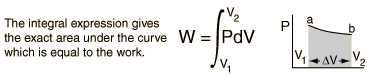# △E….LOL

Following up my last post I would like to go a step further by talking about the conservation of energy and the definition of enthalpy. What is another way to derive △E?

Another way to derive △E for the system is from LOL diagrams as done in physics. If you do not know what LOL diagrams are, please read the following blog that does a great job of explaining the concept from my former physics teacher:

http://kellyoshea.wordpress.com/2012/03/05/energy-bar-charts-lol-diagrams/

The first law of thermodynamics is the application of the conservation of energy principle to heat and thermodynamic processes. Several equations come to mind when looking at this concept:

∆H = ∆E + ∆PV (from definition) and ∆E = q + w. For example, at constant pressure, q = ∆H.

The change in internal energy of a system is equal to the heat added to the system minus the work done by the system. In the context of chemical reactions and process, it may be more common to deal with situations where work is done on the system rather than by it.

Four quantities called “thermodynamic potentials” are useful in the chemical thermodynamics of reactions and non-cyclic processes. They are internal energy, the enthalpy, the Helmholtz free energy and the Gibbs free energy. Enthalpy is defined by

H = E + PV

P is your pressure, V is your volume, and E is your internal energy. The internal energy E might be thought of as the energy required to create a system in the absence of changes in temperature or volume. But if the process changes the volume, as in a chemical reaction which produces a gaseous product, then work must be done to produce the change in volume. For a constant pressure process the work you must do to produce a volume change ΔV is PΔV. Then the term PV can be interpreted as the work you must do to “create room” for the system if you presume it started at zero volume.

When work is done by a thermodynamic system, it is ususlly a gas that is doing the work. The work done by a gas at constant pressure is:For non-constant pressure, the work can be visualized as the area under the pressure-volume curve which represents the process taking place. The more general expression for work done is:Work done by a system decreases the internal energy of the system, as indicated in the First Law of Thermodynamics.Understanding how the conservation of energy, work, and enthalpy function we learn a new way to find △E from LOL diagrams or given data. We can manipulate the various formulas in order to solve for △E, our system’s internal energy. When we look at an lol diagram, we can find out what we need to put in the various formulas in order to manipulate them.

-Nnana Amakiri

References:

http://web.mit.edu/16.unified/www/FALL/thermodynamics/thermo_4.htm

http://hyperphysics.phy-astr.gsu.edu/hbase/thermo/firlaw.html

http://kellyoshea.wordpress.com/2012/03/05/energy-bar-charts-lol-diagrams/